# Utility Functions for Survival Analysis

Functional programming principles to iteratively run Cox regression and plot its results. The results are reported in tidy data frames. Additional utility functions are available for working with other aspects of survival analysis such as survival curves, C-statistics, etc.

This package uses functional programming principles to iteratively run Cox regression and plot its results. The results are reported in tidy data frames. Additional utility functions are available for working with other aspects of survival analysis such as survival curves, C-statistics, etc. It has the following features (grouped by major topics):

Cox Regression

• `get_cox_res`: Run univariate or multivariate cox regression.
• `iter_get_cox_res`: Wrapper over `get_cox_res` to faciliate ease of multiple `get_cox_res` runs. Internally, this makes use of `purrr:map` to iterate over a list of features.
• `plot_cox_res`: Generates a forest plot of the univariate or multivariate cox regression results from `get_cox_res`.

Kaplan Meier Estimates/Curves

• `get_surv_prob`: Calculates the survival probability at specified times from a survival curve.
• `get_nrisk_tbl`: Provides a number at risk table as typically seen in publications.
• `get_logrank_res`: Runs a log-rank test.

Other

• `get_c_stat`: Calculate C-statistics.

# How to Install

To get the released version from CRAN:

You can also get survutils through conda:

To install the latest developmental version from github:

# Cox Regression

`survutils` provides a nice wrapper function `get_cox_res` that allows you to quickly run an univariate or multivariate cox regression on a set of data. The input data is a data.frame for instance (taking the colon dataset from the `survival` R package as the example):

age obstruct time status rx
43 0 1521 1 Lev+5FU
43 0 968 1 Lev+5FU
63 0 3087 0 Lev+5FU
63 0 3087 0 Lev+5FU
71 0 963 1 Obs
71 0 542 1 Obs

The relevant columns are:

• `age` and `obstruct`: These are the features we want to regress on.
• `time`: Time to event.
• `status`: Event status (1 for event; 0 for non-event).
• `rx`: Different treatment groups.

Then to run `get_cox_res`:

term estimate std.error statistic p.value conf.low conf.high test_type
age 0.9983432 0.0028040 -0.5913434 0.5542904 0.9928717 1.003845 multicox
obstruct 1.2677379 0.0808045 2.9359039 0.0033258 1.0820531 1.485287 multicox

This runs a multivariate cox regression on the entire set of data. We can plot the results using `plot_cox_res`: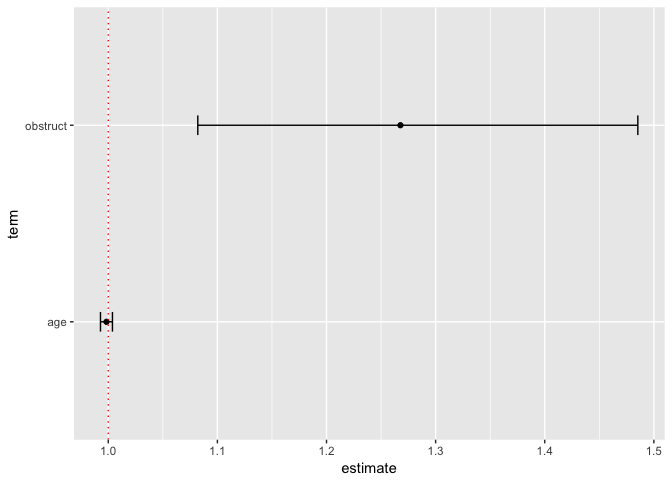This gives us a forest plot with the hazard ratio and confidence evidence for each feature. If we are interested in running cox regression within each treatment group, we can make use of the `group` parameter.

group term estimate std.error statistic p.value conf.low conf.high test_type
Obs age 1.0026174 0.0046032 0.5678581 0.5701313 0.9936124 1.0117040 multicox
Obs obstruct 1.2123725 0.1319705 1.4592591 0.1444938 0.9360576 1.5702528 multicox
Lev age 1.0042268 0.0048754 0.8651343 0.3869651 0.9946764 1.0138689 multicox
Lev obstruct 1.4151910 0.1293943 2.6837694 0.0072797 1.0981822 1.8237097 multicox
Lev+5FU age 0.9869403 0.0051866 -2.5345800 0.0112582 0.9769584 0.9970242 multicox
Lev+5FU obstruct 1.0844978 0.1677669 0.4835103 0.6287334 0.7805940 1.5067186 multicox

Notice how the output data.frame now has cox regression results for each treatment group (i.e. Obs, Lev, Lev+5FU). We can use the `plot_cox_res` function again and pass in a `facet.formula` to plot these results very easily: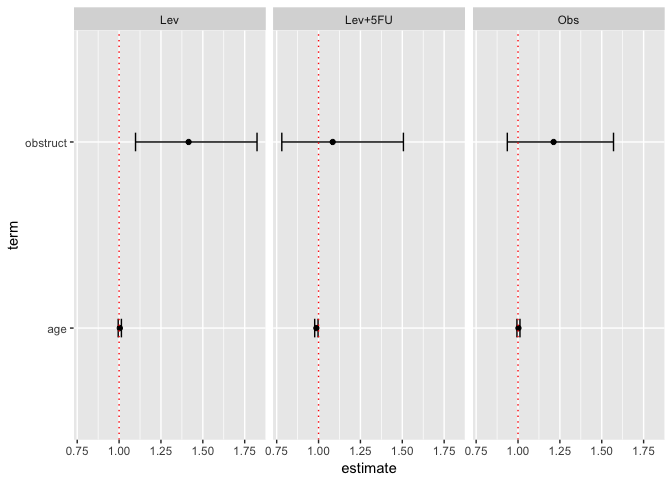This will facet the groups (per column) so that we can visualize the cox regression results for each treatment group. The formula is the format for `ggplot2::facet_grid` with the full documentation listed here. In short, the left hand side of the formula indicates what you want to facet by row. The right hand side of the formula indicates what you want to facet by column. By specifically `. ~ group`, we are indicating we do not want to facet by row (this is indicated by the `.`) and we want to facet the `group` variable by column.

We could have facetted by row too very easily: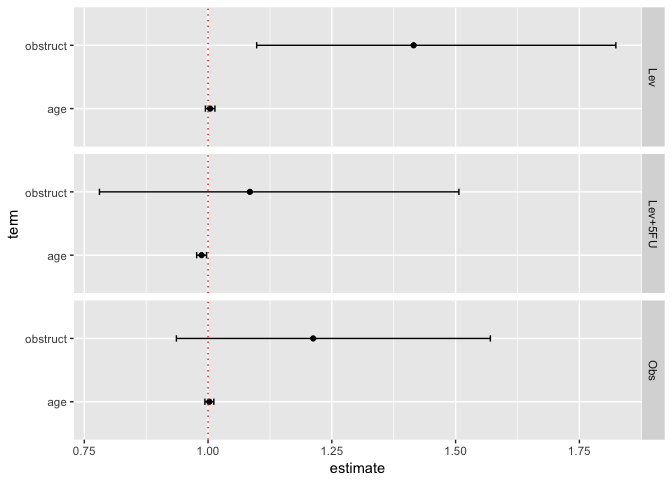There are also other options (see `?plot_cox_res` for full options) such as the ability to add colors: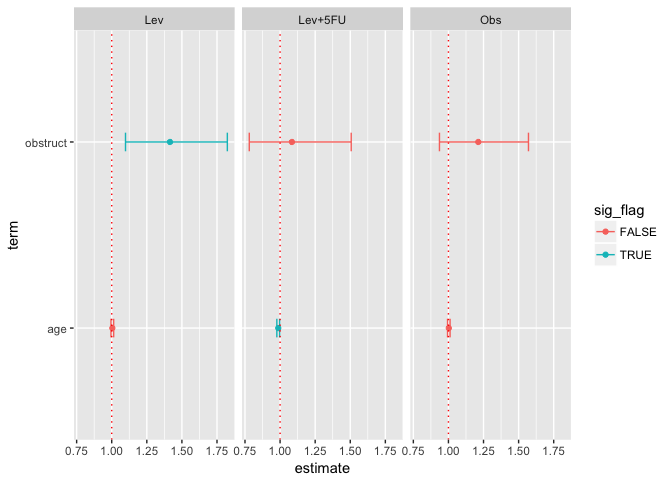# Running Cox Regression Multiple Times

One useful function is the `iter_get_cox_res` which allows you to easily run the `get_cox_res` function multiple times without needing to setup a for loop yourself. This is useful in situations where you might need to perform multiple pairwise multivariate Cox regression analysis to test the independence of a novel prognostic biomarker to existing biomarkers.

The input to the `iter_get_cox_res` function is the same as `get_cox_res` with the only exception being the features parameter which takes a list of vectors. Each element in the list indicates the features you want to perform Cox regression on:

The output is a data frame with a `iter_num` column indicating a separate Cox regression result from `get_cox_res`:

iter_num term estimate std.error statistic p.value conf.low conf.high test_type
1 age 0.9983432 0.0028040 -0.5913434 0.5542904 0.9928717 1.003845 multicox
1 obstruct 1.2677379 0.0808045 2.9359039 0.0033258 1.0820531 1.485287 multicox
2 age 0.9975589 0.0027949 -0.8744983 0.3818469 0.9921094 1.003038 unicox

One could plot then the multiple Cox regression with facet by row as follows: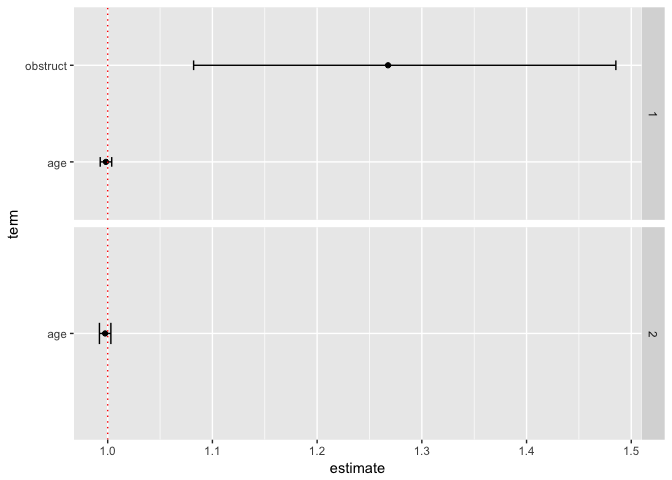By default, all features will appear in each facet. The `facet.scales` parameter drops features on the y-axes that are not part of the specific Cox regression.

You can even combine this with the group parameter:

iter_num group term estimate std.error statistic p.value conf.low conf.high test_type
1 Obs age 1.0026174 0.0046032 0.5678581 0.5701313 0.9936124 1.0117040 multicox
1 Obs obstruct 1.2123725 0.1319705 1.4592591 0.1444938 0.9360576 1.5702528 multicox
1 Lev age 1.0042268 0.0048754 0.8651343 0.3869651 0.9946764 1.0138689 multicox
1 Lev obstruct 1.4151910 0.1293943 2.6837694 0.0072797 1.0981822 1.8237097 multicox
1 Lev+5FU age 0.9869403 0.0051866 -2.5345800 0.0112582 0.9769584 0.9970242 multicox
1 Lev+5FU obstruct 1.0844978 0.1677669 0.4835103 0.6287334 0.7805940 1.5067186 multicox
2 Obs age 1.0019378 0.0045949 0.4213225 0.6735196 0.9929551 1.0110018 unicox
2 Lev age 1.0032152 0.0048522 0.6615663 0.5082492 0.9937198 1.0128013 unicox
2 Lev+5FU age 0.9866753 0.0051580 -2.6006591 0.0093045 0.9767506 0.9967007 unicox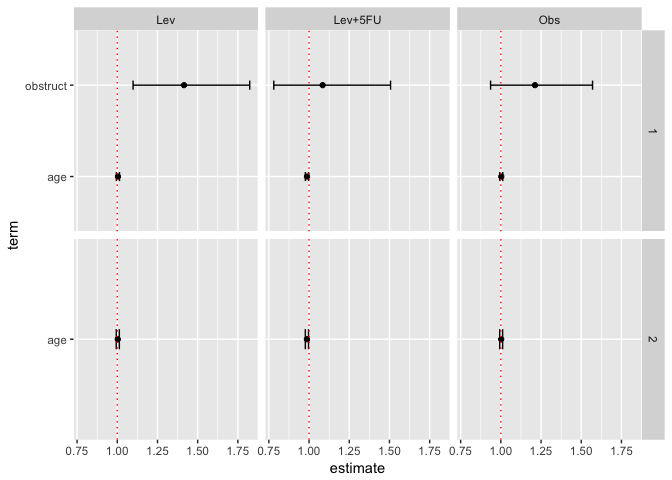# Kaplan Meier Estimates/Curves

If you have generated a Kaplan-meier, there are several functions you can use to retrieve important statistics. For example, the `get_surv_prob` function is used for retrieving survival probability at certain times. Here is an example of how to generate survival probabilities for just the “Obs” arm at times 100, 200, and 300:

Here is a small trick you can employ to get the survival probability that for both arms simultaneously:

surv_prob_time group surv_prob
100 Obs 0.9730159
200 Obs 0.9174603
300 Obs 0.8476190
100 Lev 0.9708942
200 Lev 0.9110224
300 Lev 0.8543053
100 Lev+5FU 0.9785720
200 Lev+5FU 0.9439177
300 Lev+5FU 0.9059629

You can also retrieve a number at risks table using the `get_nrisk_tbl` function. Here we will use it to get the number at risk at time 100, 200, and 300:

strata time n.risk
rx=Obs 100 613
rx=Obs 200 578
rx=Obs 300 534
rx=Lev 100 601
rx=Lev 200 563
rx=Lev 300 528
rx=Lev+5FU 100 593
rx=Lev+5FU 200 572
rx=Lev+5FU 300 549

# survutils 1.0.2

Patch to fix test failures

Latest version of broom (0.5.0) returns tibbles now. As the `get_cox_res` function uses broom, the results were now being returned as tibbles. The `test_get_cox_res.R` uses `expect_equal` to test whether two data frames are equivalent, but this does not work for tibbles. This patch changes it such that the test first converts the tibbles to data frames to allow `expect_equal` to work.

# survutils 1.0.1

• Adding a unit testing framework (#9)
• Add check for whether endpoint and endpoint.code input values were swapped (#7)
• Fix "match.arg(broom.fun) : 'arg' should be one of “tidy”, “glance”" error when running get_cox_res() (#11)
• Add default significance line for plot_cox_res (#11)

# survutils 1.0.0

• First major release of survutils

# Reference manual

install.packages("survutils")

1.0.2 by Fong Chun Chan, 2 years ago

https://github.com/tinyheero/survutils

Report a bug at https://github.com/tinyheero/survutils/issues

Browse source code at https://github.com/cran/survutils

Authors: Fong Chun Chan [aut, cre]

Documentation:   PDF Manual